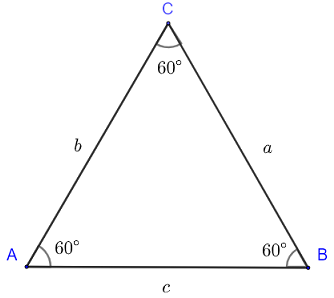# Triangles

Definitions and properties of triangles in geometry are presented.

## Angles of a Triangle

A triangle is a flat and closed two-dimensional figure with three (straight) sides. The triangle has three interior angles ∠A, ∠B and ∠C shown in the figure below, three vertices A, B and C and three sides a, b and c.Note that the angles may take the names of the vertices.
The sum of all three interior angles of a triangle is equal to 180�:      ∠A + ∠B + ∠C = 180�
The perimeter P of a triangle is equal to the sum of all three sides of the triangle:      P = a + b + c.
Several
formulas of the area of a triangle are also included.
More
triangle problems are included.

## Isosceles Triangle

An isosceles triangle has two congruent sides and therefore also two congruent angles. An isosceles triangle ABC is shown in the figure below.Important Properties of Isosceles Triangles
1) In the above triangle sides AC = b and BC = a are congruent and therefore a = b.
2) Angles A and B are also congruent and therefore ∠A = ∠B.
3) CH is the
altitude, median and angle bisectors from the vertex C.
3) CH splits the isosceles triangle ABC into two congruent right triangles: ACH and BCH.
More
isosceles triangle problems are included.

## Equilateral Triangle

An equilateral triangle has all three sides congruent and all three angles congruent.Important Properties of Isosceles Triangles
1) In the above triangle, all three sides AC = b, BC = a and AB = c are congruent and therefore a = b = c.
2) All A, B and C are congruent and therefore ∠A = ∠B = ∠B = 60�.
3) Any segment drawn from a vertex and perpendicular to the base opposite that vertex is the
altitude, median and angle bisectors from that vertex.
3) Any median splits the triangle ABC into two congruent right triangles.
More
equilateral triangle problems are included.

## Right Triangle

A right triangle has one (right) angle whose size is equal to 90�.The side BA, opposite the right angle C, is called the hypotenuse, CA and CB are called the sides of the right triangle ABC.
More
questions and problems on right triangles are included.

### Pythagorean Theorem

In any right triangle with hypotenuse c and sides a and b, the Pythagorean theorem may be used to write:

c 2 = a 2 + b 2

## Sine law in a Triangle

Triangles without a right angle may be solved using sine law.
The following notations are used: AB = c opposite angle C, BC = a opposite angle A and CA = b opposite angle B.The
sine law is expressed as follows:

a / sin(A) = b / sin(B) = c / sin(C)

## Cosine law in a Triangle

The cosine law may also be used to solve triangles without right angle. The cosine law is expressed as follows:

a 2 = b 2 + c 2 - 2 b c cos(A)

b 2 = a 2 + c 2 - 2 a c cos(B)

c 2 = b 2 + a 2 - 2 b a cos(C)

## More References and Links to Triangles

Triangle Problems
Questions on Angles with Solutions and Explanations
Geometry Tutorials and Problems
The Four Pillars of Geometry - John Stillwell - Springer; 2005th edition (Aug. 9 2005) - ISBN-10 : 0387255303
Geometry: A Comprehensive Course - Daniel Pedoe - Dover Publications - 2013 - ISBN: 9780486131733
Geometry: with Geometry Explorer - Michael Hvidsten - McGraw Hill - 2006 - ISBN: 0-07-294863-9